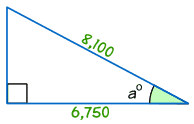# find the missing angle

These are the four steps we need to follow:

• Step 1 Find which two sides we know â€“ out of Opposite, Adjacent and Hypotenuse.
• Step 2 Use SOHCAHTOA to decide which one of Sine, Cosine or Tangent to use in this question.
• Step 3 For Sine calculate Opposite/Hypotenuse, for Cosine calculate Adjacent/Hypotenuse or for Tangent calculate Opposite/Adjacent.
• Step 4 Find the angle from your calculator, using one of sin-1, cos-1 or tan-1

### Example

Find the size of angle a0• Step 1 The two sides we know are Adjacent (6,750) and Hypotenuse (8,100).
• Step 2 SOHCAHTOA tells us we must use Cosine.
• Step 3 Calculate Adjacent / Hypotenuse = 6,750/8,100 = 0.8333
• Step 4 Find the angle from your calculator using cos-1 of 0.8333:

cos a0 = 6,750/8,100 = 0.8333

cos-1 of 0.8333 = 33.60 (to 1 decimal place)

Example #1

Find the missing angle when you are given the following dimensions.

BC = 3; AC = 16; AB = 8, Cos ? = 3/16Explanation

The missing value is x than Cos 3 / 16

Cos x = 0.2

x = Cos-1 0.2

x = 78.5

Practice Problem #1:

Find the missing angle:

BC = 10 ; AC = 18 ; AB = 7, Sin__?__ = 7 / 18

Explanation #1

The missing value is x than Sin 7 / 18

Sin x = 0.4

x = Sin-1 0.4

x = 23.60

Practice Problem #2)

Find the missing angle:

BC = 24 ; AC = 13 ; AB = 4, tan_?___ = 13 / 24

Explanation #2

The missing value is x than tan 13 / 24

tan x = 0.5

x = tan-1 0.5

x = 26.60

Practice Problem #3)

Find the missing angle:

BC = 18 ; AC = 33 ; AB = 15, Cos__?__ = 18 / 33

Explanation #3

The missing value is x than Cos 18 / 33

Cos x = 0.5

x = Cos-1 0.5

x = 600

Review

Directions: Find the missing angle in each of the following problems.

1. QR = 36 ; PR = 45 ; PQ = 21, Sin __?___ = 21 / 45

2. QR = 16 ; PR = 2 ; PQ = 8, tan __?__ = 8 / 16

3. QR = 15 ; PR = 28 ; PQ = 15, Cos __?__ = 15 / 28

4. QR = 47 ; PR = 64 ; PQ = 38, tan __?__ = 38 / 47

5. QR = 4 ; PR = 17 ; PQ = 12, Sin __?___ = 12 / 17

6. QR = 36 ; PR = 59 ; PQ = 20, Cos __?___ = 36 / 59

7. QR = 82 ; PR = 63 ; PQ = 29, tan __?__ = 29 / 82

8. QR = 10 ; PR = 28 ; PQ = 8, Sin __?___ = 8 / 28

9. QR = 51 ; PR = 42 ; PQ = 9, tan __?__ = 9 / 51

10. QR = 16 ; PR = 20 ; PQ = 17, Cos __?___ = 16 / 20

11. QR = 12 ; PR = 84 ; PQ = 60, Sin __?_ = 60 / 84

12. QR = 19 ; PR = 32 ; PQ = 45, Cos __? = 19 / 32

13. QR = 76 ; PR = 27 ; PQ = 64, tan __ ?_ = 64 / 76

14. QR = 26 ; PR = 48 ; PQ = 37, Cos __?_ = 37 / 48

15. QR = 19 ; PR = 66 ; PQ = 23, Cos __?__= 23 / 66